### Centimeter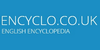10-2 meter.

### Centimeter• (n.) Alt. of Centimetre
Found on http://thinkexist.com/dictionary/meaning/centimeter/

### centimeter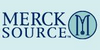(cm) (sen´tĭ-me″tәr) one hundredth (10−2) of a meter. cubic centimeter(cm3) (cc) a unit of capacity, being that of a cube each side of which measures 1 cm; equal to 1 mL.
Found on http://www.encyclo.co.uk/local/21001

### centimeter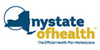(cm) A centimeter is one one-hundredth (1/100) of a meter. One inch is about 2.5 cm; a penny is about 2 cm wide.
Found on https://www.health.ny.gov/environmental/glossary/

### centimetercentimetre noun a metric unit of length equal to one hundredth of a meter
Found on https://www.encyclo.co.uk/local/20974

### centimeter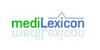(cm) Type: Term Pronunciation: sen′ti-mē′ter Definitions: 1. One hundredth of a meter; 0.3937008 inch.
Found on http://www.medilexicon.com/medicaldictionary.php?t=16123

### Centimeter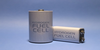A centimetre (American spelling: centimeter, symbol cm) is a unit of length in the metric system, equal to one hundredth of a metre, which is the current SI base unit of length.
Found on http://en.wikipedia.org/wiki/Glossary_of_fuel_cell_terms

### centimeter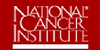A measure of length in the metric system. There are 100 centimeters in a meter and 2
Found on http://www.cancer.gov/dictionary?expand=C

### Centimeter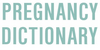A unit of length in the metric system that is often used to determine cervical dilation. The cervix will begin at a tightly closed 0 cm and will open to a fully dilated, 10 cm, in which pushing can begin.
Found on http://www.pregnology.com/Pregnancy-dictionary/

### centimeter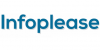centimeter (sen'timē"tur) , abbr. cm, unit of length equal to 0.01 meter, the basic unit of length in the metric system. The centimeter is the unit of length in the cgs system. It is approximately equal to 0.39 inch, or 1 inch equals about 2.54 centimeters. See weights and measures.One hundredth of a meter; 0.3937008 inch. ... Cubic centimeter (cc, c.c.), one thousandth of a liter; 1 milliliter. ... Abbreviation: cm ... (05 Mar 2000) ...[n] - a metric unit of length equal to one hundredth of a meter